# Data Validation with Specific Year, Month, Day or Excel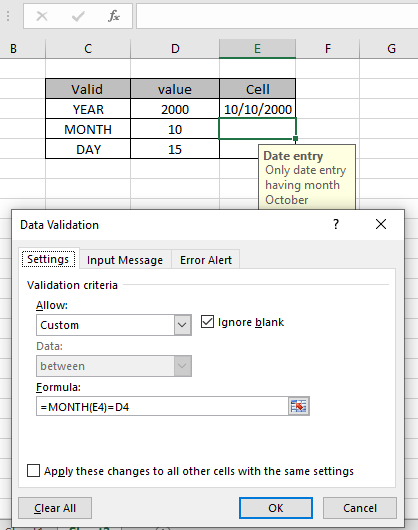In this article, we will learn how to validate data entries that must contain specific year, specific month or specific date using data validation in excel. In other words, you can make sure that excel only excepts a date if it from defined year, month or date, after learning this data validation formula.

How to solve the problem?

To allow only specified kind of data, we will use these functionalities and functions of Excel.

Data Validation date in Specific year

We will construct a formula out of it. First the date entry will be checked for the specific year using the YEAR function.

Use the formula in Data Validation tool

= YEAR (cell) = year_value

cell : cell value where specific date entry to be checked

year_value : entry to be checked with specific year value

Example:

All of these might be confusing to understand. So, let's test this formula via running it on the example shown below. Here we will apply data validation on the cell to restrict the user to input any value which doesn't satisfy the mentioned condition.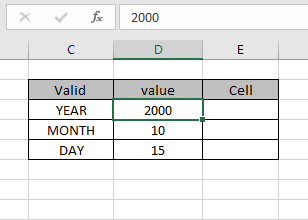Assume the cell be E3 cell and specific year be 2000 to check. We need to lock this input cell with a formula in the data validation tool. Now we learn how to do it. Follow these steps to do the same:

• Select the cells where data validation need to apply.
• Click Data > Data Validation option from the menu.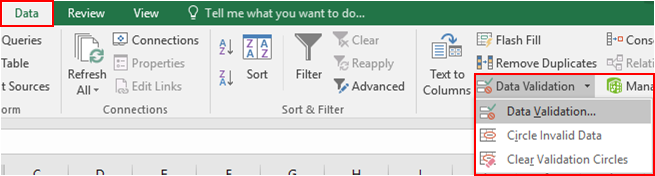• The Data validation dialog box appears on the front.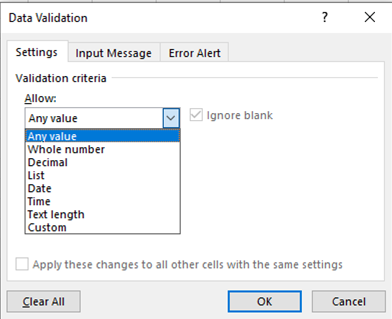• In the Settings option, Select the Custom option from the Allow list.
• The formula box appears under the same option.
• Use the formula in the formula box.
= YEAR (E3) = D3

Explanation:

• YEAR function extracts year value from the input value in E3 cell and returns the year value.
• The return year value to be checked with the given value in D3 cell which is 2000.
• If the formula returns TRUE, then the cell accepts the value or else it returns an error message.

The formula looks like as shown in the image below.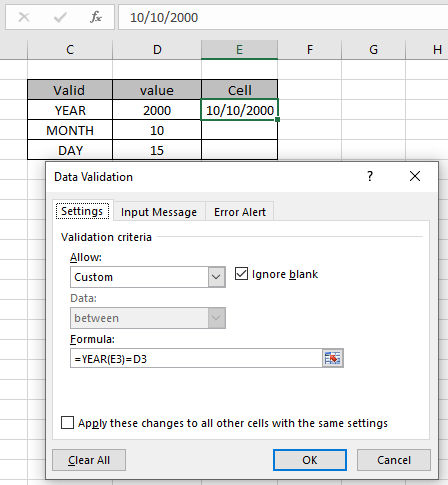The value to check and to check with are given as cell reference. As I entered a date value which doesn't satisfy the condition.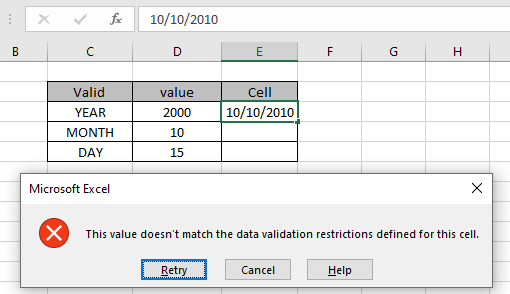As you can see an error is generated "This value doesn't match the data validation restrictions defined for this cell". Now when I enter the value as shown below which the cell accepts.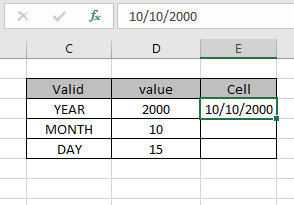But now the problem is how the user knows what restrictions are there and what type of value the cell can accept. Now you can customize the error alert and input message to let the user know what the cell accepts.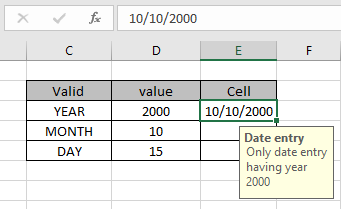The input message display as shown above.

Data Validation date in Specific Month

We will construct a formula out of it. First the date entry would be checked for the specific Month using the MONTH function.

Use the formula in Data Validation tool

= MONTH (cell) = month_value

cell : cell value where specific date entry to be checked
month_value : entry to be checked with specific month valuePerform the same task for the Specific month as shown above. Display input message and error alert.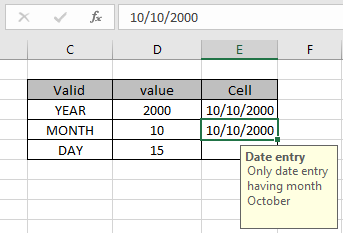As you can see, the cell accepts the right date entry.

Data Validation date in Specific day

We will construct a formula out of it. First the date entry would be checked for the specific date using the MONTH function.

Use the formula in Data Validation tool

= DAY (cell) = day_value

cell : cell value where specific date entry to be checked
day_value : entry to be checked with specific day value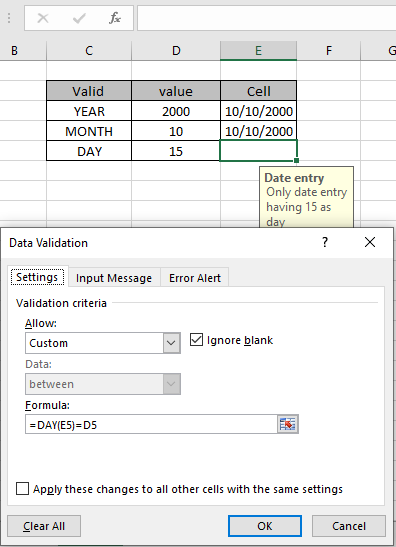Perform the same task for the Specific month as shown above. Display input message and error alert.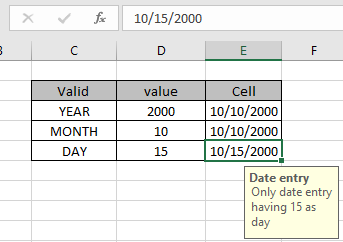As you can see, the cell accepts the right date entry.

Here are all the observational notes regarding using the formula.

Notes:

1. Apply the data validation tool to restrict the user to input invalid value which doesn't satisfy the condition.
2. Input date value must be in valid date format or else the cell doesn't accept the input value.
3. Formulas inside the data validation tool can be customized.
4. YEAR, MONTH & DAY function extracts the year, month or day value from the valid input date value.
5. Operators like equals to ( = ), less than equal to ( <= ), greater than ( > ) or not equals to ( <> ) can be performed within function applied with numbers only.

Hope this article about how to do Data validation with specific year, month, day in excel is explanatory. Find more articles on Date & Time functions here. If you liked our blogs, share it with your friends on Facebook. And also you can follow us on Twitter and Facebook. We would love to hear from you, do let us know how we can improve, complement or innovate our work and make it better for you. Write us at info@exceltip.com

Related Articles:

Data Validation in Excel : restricts user to input value manually in Excel.

Way to use Vlookup function in Data Validation : allow values from the vlookup table in Excel.

Restrict Dates using Data Validation : allow dates in the cell which lays within Excel date format.

How to give the error messages in Data Validation : customize your data validation cell with error messages.

Create Drop Down Lists in Excel using Data Validation : restricts users to input data manually.

Popular Articles:

50 Excel Shortcut to Increase Your Productivity : Get faster at your task. These 50 shortcuts will make you work even faster on Excel.

How to use the VLOOKUP Function in Excel : This is one of the most used and popular functions of excel that is used to lookup value from different ranges and sheets.

How to use the COUNTIF function in Excel : Count values with conditions using this amazing function. You don't need to filter your data to count specific values. Countif function is essential to prepare your dashboard.

How to Use SUMIF Function in Excel : This is another dashboard essential function. This helps you sum up values on specific conditions.

Terms and Conditions of use

The applications/code on this site are distributed as is and without warranties or liability. In no event shall the owner of the copyrights, or the authors of the applications/code be liable for any loss of profit, any problems or any damage resulting from the use or evaluation of the applications/code.